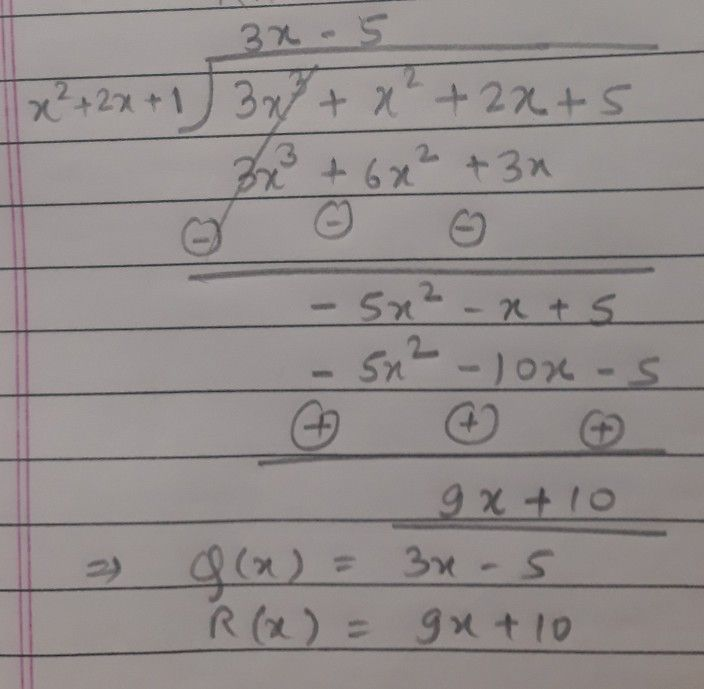Symbol
Problem$3x-5$ $3$ $2$ $2+2x+1\right)3-$ $x$ $+$ $x$ $+$ $2$ $x$ $+5$ $3x^{3}+6x^{2}+3x$ $=$ $→$ $-5x^{2}-x+5$ $-5x^{2}-10x-5$ $+$ $+$ $-$ $+$ $9x+10$
1st-6th grade
Probability and Statistics
SolutionQanda teacher - Sid711